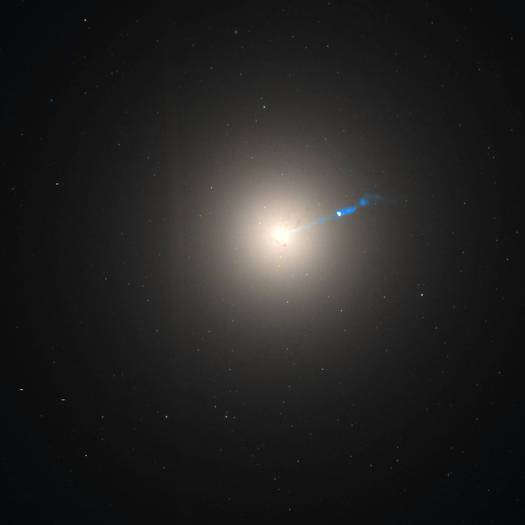# Determining the Mass of Elliptical Galaxies by Velocity Dispersion Method#### Image contains the galactic core of a galaxy - Messier 87 (M87) or NGC4486 as seen by the Hubble Space Telescope with its blue plasma jet clearly visible (composite image of observations in visible and infrared light). Image © NASA, ESA and the Hubble Heritage Team (STScI/AURA).

M87 is an elliptical (E0) galaxy - "E0" designates an elliptical galaxy that displays no flattening - that is, it appears spherical. Sometimes this galaxy is categorized as E0p - "p" indicating a peculiar galaxy that does not fit cleanly into the classification scheme; in this case, the peculiarity is the presence of the jet emerging from the core- considerably a supermassive black hole.

Measurement of velocity dispersion of stars in the nuclear region with the help of high dispersion spectrograms serves the purpose of estimating the total mass of elliptical galaxies. In this method, all the stars mass is assumed to be same, so we can apply Virial Theorem. In order to obtain the stellar velocity dispersion from the composite spectrum, one assumes a particular model of velocity dispersion law like the Gaussian law and fits the observed line profiles with the experimental line profiles in the laboratory. The dispersion of particles with which the experimental profiles best fit with the observed profiles is considered to be the required dispersion of stars.

However, the method is quite difficult and may involve significant uncertainty, this method is widely used by astronomers in the case of elliptical galaxies.

When the radial velocity dispersion of stars is obtained, the value is multiplied by some suitable numerical factor in order to realize the total velocity dispersion of stars in the galaxy. Then obtained average of the space velocities of stars is denoted by ㄑ𝒗2½

and the standard Virial Theorem is 2T + U = 0.

where T is the total kinetic energy of a self-gravitating body due to the motions of its constituent parts and U is the gravitational potential energy.

Assuming the same mass of stars, this will give us a relation;

Mㄑ𝒗2 〉 = 0

Where

U = -G ∫ M(r)/r dM

(Integration is from 0 to R)

Mis the total mass of the galaxy, R the distance of its periphery from the centre and ㄑ𝒗2½ the average space velocity referred to ist centre of mass.

Now here in order to evaluate U, one must have to assume some mass distribution law of the galaxy. Since the light distribution in the galaxy can be measured photometrically, it has been customary to assume the mass distribution to be identical with the measured light distribution and that the mass of light ratio is constant. Thus knowingㄑ𝒗2 and U, we can calculate the total mass of a galaxy from the above first equation.

In simpler language, the elliptical galaxy's mass = k × (velocity dispersion)2 × (the distance the stars are from the galaxy centre)/G, where k is a factor that depends on the shape of the galaxy and the angle the galaxy is from Earth.